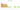# CHF

## Swiss Franc

#### how has the value of the currency changed in the last year?## USD 1 = CHF 0.92339

How much is10 US dollars worth inSwiss francs?
At the current exchange rate, 10 US dollars is worth 9.23 Swiss francs
How much is50 US dollars worth inSwiss francs?
At the current exchange rate, 50 US dollars is worth 46.17 Swiss francs
How much is100 US dollars worth inSwiss francs?
At the current exchange rate, 100 US dollars is worth 92.34 Swiss francs
How much is500 US dollars worth inSwiss francs?
At the current exchange rate, 500 US dollars is worth 461.7 Swiss francs
How much is2,000 US dollars worth inSwiss francs?
At the current exchange rate, 2,000 US dollars is worth 1,846.78 Swiss francs
How much is10 US dollars worth inSwiss francs?
At the current exchange rate, 10 US dollars is worth 9.23 Swiss francs
How much is50 US dollars worth inSwiss francs?
At the current exchange rate, 50 US dollars is worth 46.17 Swiss francs
How much is100 US dollars worth inSwiss francs?
At the current exchange rate, 100 US dollars is worth 92.34 Swiss francs
How much is500 US dollars worth inSwiss francs?
At the current exchange rate, 500 US dollars is worth 461.7 Swiss francs
How much is2,000 US dollars worth inSwiss francs?
At the current exchange rate, 2,000 US dollars is worth 1,846.78 Swiss francs